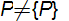# Write which of the following statements are true?`
Question:

(i) The set of all integers is contained in the set of all set of all rational numbers.

(ii) The set of all crows is contained in the set of all birds.

(iii) The set of all rectangle is contained in the set of all squares.

(iv) The set of all real numbers is contained in the set of all complex numbers.

(v) The sets P = {a} and B = {{a}} are equal.\

(vi) The sets $A=\{x: x$ is a letter of the word "LITTLE" $\}$ and,

$B=\{x: x$ is a letter of the word "TITLE" $\}$ are equal.

Solution:

(i) True

A rational number is any $\frac{m}{n}$, where $m$ and $n$ are any integers $(n \neq 0)$. Any integer can be put into that form by setting $n=1$. Therefore, the set of all integers is contained in the set of all rational numbers.

(ii) True
All crows are birds. Therefore, the set of all crows is contained in the set of all birds.
(iii) False
Every square can be a rectangle, but every rectangle cannot be a square.
(iv) True

Every real number can be written in the (bi) form. Thus, we can say that the set of all real numbers is contained in the set of all complex

numbers.

(v) False

= {a}

= {{a}} = {P}(vi) True
We have:

{x:x is a letter of the word LITTLE} = {L, I, T, E}

B = {x:x is a letter of the word TITLE} = {T, I, L, E}

Sets A & B are equal because every element of A is a member of B & every element of B is a member of A.